# Precalculus : Graph a Parabola

## Example Questions

### Example Question #1 : Parabolas

Which of the following could be the graph of f(x)?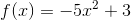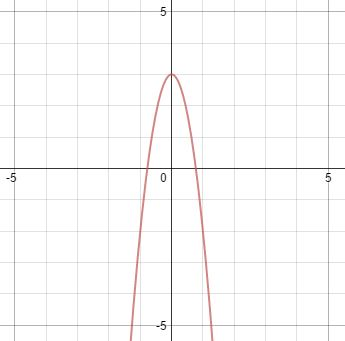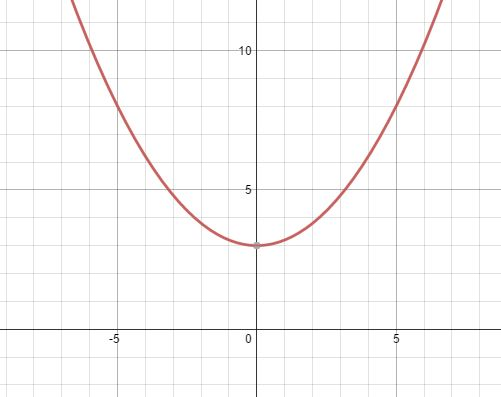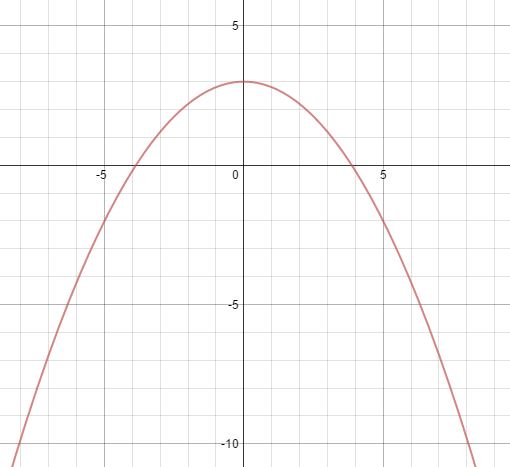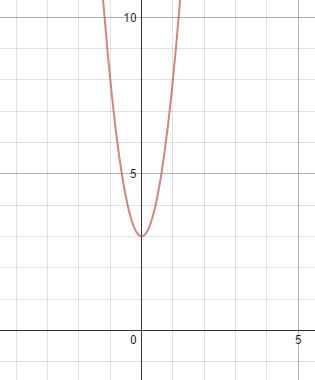Explanation:

Which of the following could be the graph of f(x)?Begin by realizing this must be a downward facing parabola with its vertex at (0,3)

We know this because of the negative sign in front of the 5, and by the constant term of 3 on the end.

This narrows our options down to 2. One is much narrower than the other, although it may seem counterintuitive, the narrower one is what we need. This is because for every increase in x, we get a corresponding increase of times 5 in y. This translates to a graph that will get to higher values of y faster than a basic parabola. So, we need the graph below. to further confirm, try to find f(1)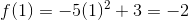So, the point (1,-2) must be on the graph, which means we must have:### Example Question #2 : Parabolas

Describe the orientation of a parabola with the following equation: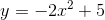Facing down

Facing to the right

Facing up

None of the other options

Facing to the left

Facing down

Explanation:

The coefficient of the squared term tells us whether the parabola faces up or down. Parabolas in general, as in the parent function, are in the shape of a U. In the equation given, the coefficient of the squared term is. Generally, if the coefficient of the squared term is positive, the parabola faces up. If the coefficient is negative, the parabola faces down. Sinceis negative, our parabola must face down.

### Example Question #3 : Parabolas

Describe the orientation of the parabola below: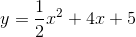Facing right

Facing down

Facing up

Facing left

None of the other options

The coefficient of the squared term tells us whether the parabola faces up or down. Parabolas in general, as in the parent function, are in the shape of a U. In the equation given, the coefficient of the squared term is. Generally, if the coefficient of the squared term is positive, the parabola faces up. If the coefficient is negative, the parabola faces down. Sinceis positive, our parabola must face up.